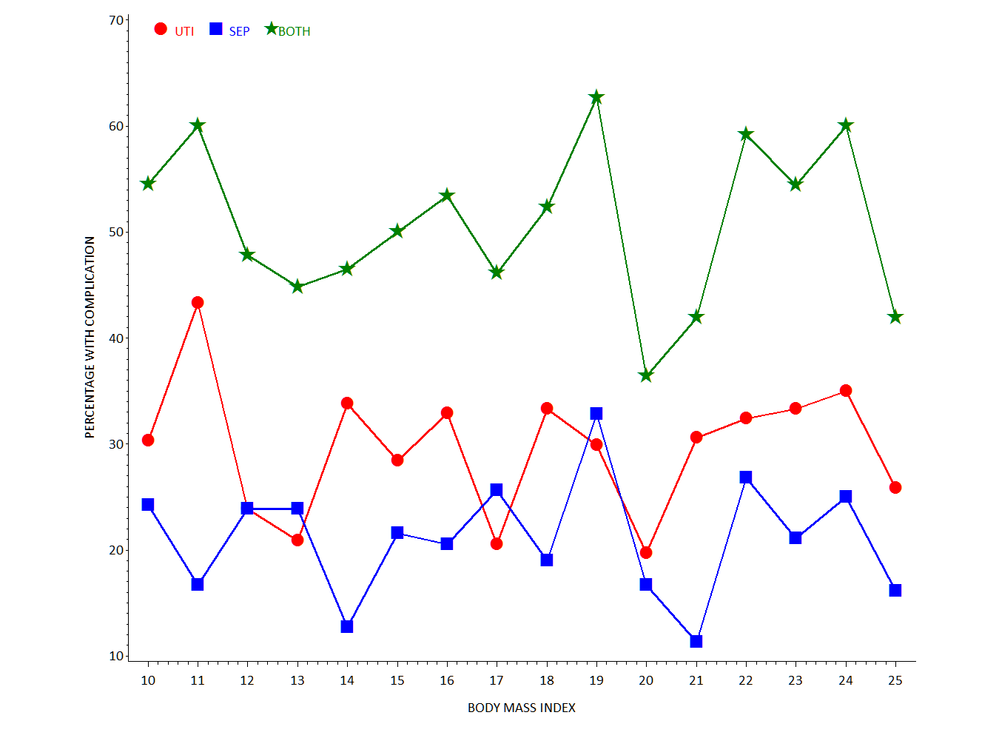## How to construct line graph of complication rate by BMI percent

This seems like such a simple thing to do, yet I am not understanding how to do this and would appreciate some help. First, I have a dataset of 1000 patients. What I am trying to do is construct a line graph of the number of patients who have urinary tract infection (y-axis) vs. BMI % (x-axis) and number of patients who have sepsis (y-axis) vs. BMI % (x-axis). The dataset has UTI (yes/no) and sepsis (yes/no) as well as a column for BMI percentage (I also have available variables giving the number of occurrences of UTI and sepsis). What I want is the rate of sepsis by BMI % and the rate of UTI by BMI %. I also want to combine sepsis and UTI and call it "complications" and then look at rate of complication by BMI %. How do I set up my data to do this and which SAS code can help me accomplish this? Like I said, this seems very simple to me, but for some reason I can't figure this out. Thanks in advance.

4 REPLIES 4

## Re: How to construct line graph of complication rate by BMI percent

I think you should simplify your question. Start with a single - clear - question and post sample data and what you'd like to see, a quick image mocked up in Excel or even hand drawn and attached would be helpful.

## Re: How to construct line graph of complication rate by BMI percent

Also with any rate you want to consider your denominator. When you say BMI, since that is likely to be a continuous measure and depending on precision of measurement and calculation method could very well have no duplicates for a sample as small as 1000.

You may need to round your BMI into useable ranges of values that make sense for the project.

And a first step would be to have your UTI and SEPSIS variables as numeric coded 1 for yes and 0 for no. Then when you take the MEAN of UTI or sepsis within any group the result is a percentage.MikeZdeb
Rhodochrosite | Level 12

## Re: How to construct line graph of complication rate by BMI percent

Hi, here's an idea using GPLOT (someone else can do SGPLOT) ...

* some fake data;
data x;
do _n_=1 to 1000;
uti = ifn(ranuni(99) lt 0.3, 1, 0);
sep = ifn(ranuni(99) lt 0.2, 1, 0);
bmi = round(10 + (15*ranuni(99)));
output;
end;
run;

* calculate percent URI and SEP within BMI groups;
proc summary data=x nway;
class bmi;
var uti sep;
output out=mydata (drop=_:) mean=;
run;

* rescale percentages (0 to 100);
data mydata;
set mydata;
uti = round(100*uti,.1);
sep = round(100*sep,.1);
comps = uti+sep;
run;

* GPLOT (someone else can do SGPLOT);
goptions reset=all ftext='calibri' htext=2 gunit=pct;

* choose some graphics options;

symbol1 i=j f='wingdings' v='6c'x h=3 c=red w=3;

symbol2 i=j f='wingdings' v='6e'x h=3 c=blue w=3;
symbol3 i=j f='wingdings' v='ab'x h=3.5 c=green w=3;

legend1 position=(top inside left) label=none offset=(3,)
value=(j=c c=red 'UTI' c=blue 'SEP' c=green 'BOTH') shape=symbol(.0001,3);

axis1 offset=(2,2)pct label=('BODY MASS INDEX');
axis2 label=(a=90 'PERCENTAGE WITH COMPLICATION');

* whitespace left/right;
title1 a=90 ls=5;
title2 a=-90 ls=5;

proc gplot data=mydata;
plot (uti sep comps) * bmi / legend=legend1 vaxis=axis2 haxis=axis1 overlay noframe;
run;
quit;ChrisNZ
Tourmaline | Level 20

## Re: How to construct line graph of complication rate by BMI percent

Something like this (untested)?

``````data HAVE;
do UTI    ='Y','N';
do SEPSIS ='Y','N';
do BMI = 0.05 to 0.95 by 0.05;
NBCASES=round(ranuni(0)*100);
output;
end;
end;
end;
run;

proc sql;
create table RATES as
select BMI
, sum(NBCASES*(UTI    ='Y'))          /sum(NBCASES) as RATE_UTI
, sum(NBCASES*(SEPSIS ='Y'))          /sum(NBCASES) as RATE_SEPSIS
, sum(NBCASES*(SEPSIS ='Y')*(UTI='Y'))/sum(NBCASES) as RATE_COMPLIC
from HAVE
group by BMI;
quit;

proc plot data=RATES;
plot (RATE_UTI RATE_SEPSIS RATE_COMPLIC)*BMI / overlay ;
format RATE: percent.;
run;
quit;``````

Discussion stats
• 4 replies
• 906 views
• 0 likes
• 5 in conversation